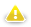## Sequence Functions

Sequence functions are functions to work with sequences in CTL.

To use sequence functions, you need a sequence in a graph. See Chapter 34, Sequences.

Sequences are accessed via `sequence()` function: specify the name of the sequence as an argument of the function.

There are three actions that can be performed on the sequence. You can get the current number of the sequence, the next number of the sequence or you may want to reset the sequence numbers to the initial number value.

WarningRemember that you should not use the functions shown below in the `init()`, `preExecute()` or `postExecute()` functions of CTL template.

See the following options:

• `sequence(<sequence name>).current()`

• `sequence(<sequence name>).next()`

• `sequence(<sequence name>).reset()`

Although these expressions return integer values, you may also want to get long or string values. This can be done in one of the following ways:

• `sequence(<sequence name>,long).current()`

• `sequence(<sequence name>,long).next()`

• `sequence(<sequence name>,string).current()`

• `sequence(<sequence name>,string).next()`

The names of the functions are self-descriptive, but there are some details useful to know:

#### Sequence Important Details

Do not use `current()` before first call of `next()`. The first call of `next()` resets the sequence to its initial value. See the example below.

There is a sequence `seq01` with 10 as an initial value and step set to 1.

```sequence(seq01).current(); // returns 10
sequence(seq01).next();    // returns 10
sequence(seq01).current(); // returns 10
sequence(seq01).next();    // returns 11
sequence(seq01).current(); // returns 11
sequence(seq01).next();    // returns 12```

Do not use sequence functions in `init()`, `preExecute()` and `postExecute()` functions of CTL template.